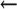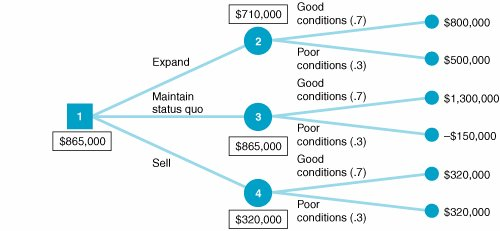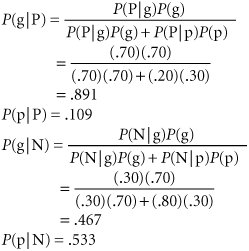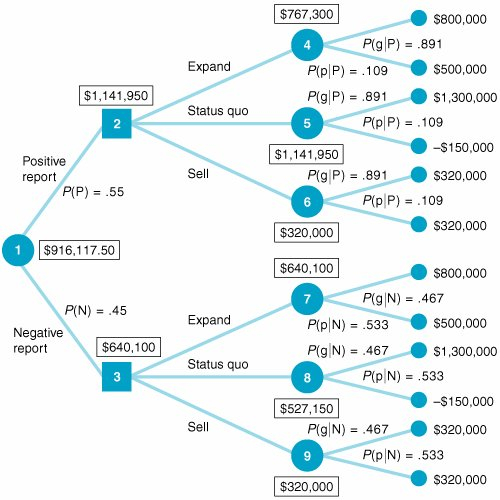# Example Problem Solution

[Page 547 ( continued )]

The following example will illustrate the solution procedure for a decision analysis problem.

#### Problem Statement

T. Bone Puckett, a corporate raider, has acquired a textile company and is contemplating the future of one of its major plants, located in South Carolina. Three alternative decisions are being considered : (1) expand the plant and produce lightweight, durable materials for possible sales to the military, a market with little foreign competition; (2) maintain the status quo at the plant, continuing production of textile goods that are subject to heavy foreign competition; or (3) sell the plant now. If one of the first two alternatives is chosen , the plant will still be sold at the end of a year. The amount of profit that could be earned by selling the plant in a year depends on foreign market conditions, including the status of a trade embargo bill in Congress. The following payoff table describes this decision situation:

[Page 548]

State of Nature

Decision

Good Foreign Competitive Conditions

Poor Foreign Competitive Conditions

Expand

\$ 800,000

\$ 500,000

Maintain status quo

1,300,000

150,000

Sell now

320,000

320,000

1. Determine the best decision by using the following decision criteria:

1. Maximax

2. Maximin

3. Minimax regret

4. Hurwicz ( a = .3)

5. Equal likelihood

2. Assume that it is now possible to estimate a probability of .70 that good foreign competitive conditions will exist and a probability of .30 that poor conditions will exist. Determine the best decision by using expected value and expected opportunity loss.

3. Compute the expected value of perfect information.

4. Develop a decision tree, with expected values at the probability nodes.

5. T. Bone Puckett has hired a consulting firm to provide a report on future political and market situations. The report will be positive (P) or negative (N), indicating either a good (g) or poor (p) future foreign competitive situation. The conditional probability of each report outcome, given each state of nature, is

P (Pg) = .70

P (Ng) = .30

P (Pp) = .20

P (Np) = .80

Determine the posterior probabilities by using Bayes's rule.

6. Perform a decision tree analysis by using the posterior probability obtained in (e).

#### Solution

Step  1.
(part A): Determine Decisions Without Probabilities

 Maximax: Expand \$ 800,000 Status quo 1,300,000Maximum Sell 320,000

Decision: Maintain status quo.

[Page 549]
 Maximin: Expand \$ 500,000Maximum Status quo -150,000 Sell 320,000

Decision: Expand.

 Minimax regret: Expand \$500,000Minimum Status quo 650,000 Sell 980,000

Decision: Expand.

 Hurwicz ( a = .3): Expand \$800,000(.3) + 500,000(.7) = \$590,000 Status quo \$1,300,000(.3) 150,000(.7) = \$285,000 Sell \$ 320,000(.3) + 320,000(.7) = \$ 320,000

Decision: Expand.

 Equal likelihood: Expand \$800,000(.50) + 500,000(.50) = \$650,000 Status quo \$1,300,000(.50) 150,000(.50) = \$575,000 Sell \$320,000(.50) + 320,000(.50) = \$320,000

Decision: Expand.

Step  2.
(part B): Determine Decisions with EV and EOL

 Expected value: Expand \$800,000(.70) + 500,000(.30) = \$710,000 Status quo \$1,300,000(.70) 150,000(.30) = \$865,000 Sell \$320,000(.70) + 320,000(.30) = \$320,000

Decision: Maintain status quo.

 Expected opportunity loss: Expand \$500,000(.70) + 0(.30) = \$350,000 Status quo \$0(.70) + 650,000(.30) = \$195,000 Sell \$980,000(.70) + 180,000(.30) = \$740,000

Decision: Maintain status quo.

Step  3.
(part C): Compute EVPI

 expected value given perfect information = 1,300,000(.70) + 500,000(.30) = \$1,060,000

[Page 550]
 expected value without perfect information = \$1,300,000(.70) 150,000(.30) = \$865,000 EVPI = \$1,060,000 865,000 = \$195,000

Step  4.
(part D): Develop a Decision TreeStep  5.
(part E): Determine Posterior Probabilities[Page 551]
Step  6.
(part F): Perform Decision Tree Analysis with Posterior ProbabilitiesIntroduction to Management Science (10th Edition)
ISBN: 0136064361
EAN: 2147483647
Year: 2006
Pages: 358

Similar book on Amazon

flylib.com © 2008-2017.
If you may any questions please contact us: flylib@qtcs.net